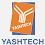## SAT Physics Conventions and Graphing - Fundamental and Derived Units

The fundamental metric units (SI units) in physics cover the basic quantities measured, such as length, mass, and time. The units measure a quantity and are given a unit name and symbol. Table 1.1 lists the fundamental quantities along with the unit names and symbols.Derived units are combinations of one or more of the fundamental units. Table 1.2 lists common derived units used in physics.Some questions on the SAT Subject Test in Physics may ask which units correctly belong to a specific quantity. An easy way to do this is to write out the principal formula for the quantity and then replace each variable on the right side of the equation with its unit symbol. There may be more than one correct answer including the unit symbol, other derived units, and fundamental units. For example, all of the following are
correct ways to express units of energy: J, N • m, and kg • m2/s2.

Derived Units
The unit of force is the newton. What are the fundamental units that make up the newton?
WHAT'S THE TRICK?
Write down the foundational formula for force.Replace the variable symbols with their matching units. Force is measured in newtons, N. Mass is measured in kilograms, kg. Acceleration is measured in meters per second squared, m/s2.
N = kg • m/s2

1.Stunning, What an Excellent post. I truly discovered this to much useful. It is the thing that I was looking for. I might want to propose you that please continue sharing such kind of data. Wholesaler of Aromatic Ingredients in UK

2.It's very nice of you to share your knowledge through posts. I am excited to read the next posts. I'm so grateful for all that you've done. Keep plugging. Keep sharing your valuable knowledge. Great blog. Weighing Scale Suppliers in UAE